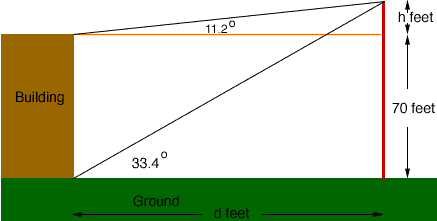Quandaries and Queries My name is Dorothy Jean and I am a 3rd year high school student. I have this problem thatI hope you can help me solve.  Thanks. From the top of a building 70ft.high, the angle of elevation of the top of the pole is 11.2 degrees. From the bottom of the building the angle of elevation of the top of the pole is 33.4 degrees. Find the (a) height of the pole and (b) the distance from the building. Hi Dorothy Jean, Her is my diagram,From the diagram  (70 + h)/d = tan(33.4o) and  h/d = tan(11.2o) Thus d = (70 + h)/tan(33.4o) = h/tan(11.2o) Solve for h. Penny Go to Math Central